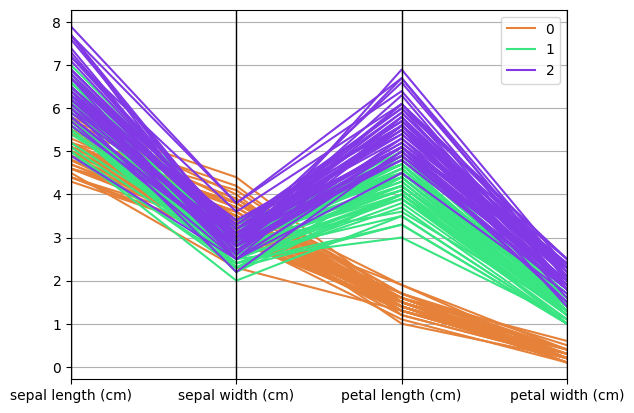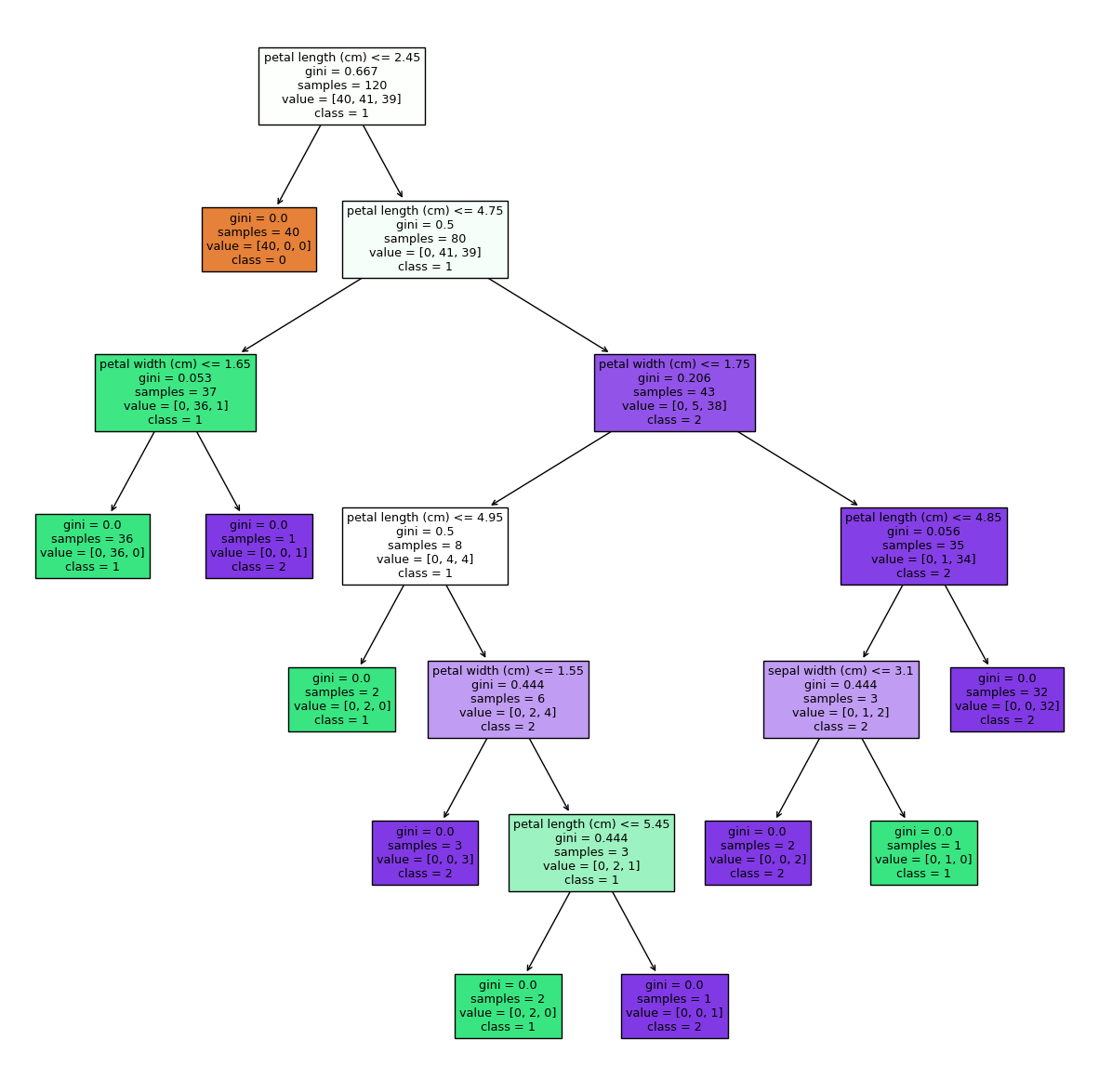Learning library

For all the self-taught geeks out there, here is our content library with most of the learning materials we have produced throughout the years.

It makes sense to start learning by reading and watching videos about fundamentals and how things work.

Data Science and Machine Learning - 16 wks

Full-Stack Software Developer - 16w

Search from all Lessons

Social & live learning

The most efficient way to learn: Join a cohort with classmates just like you, live streams, impromptu coding sessions, live tutorials with real experts, and stay motivated.

← Back to Lessons
Edit on Github
Open in Collab

# Exploring Decision Trees

## Decision trees in Python¶

Next we will see how we can implement this model in Python. To do so, we will use the scikit-learn library.

### Decision trees for classification¶

To exemplify the implementation of a classification tree we will use a dataset with few instances and which has been previously treated with a full EDA.

#### Step 1. Reading the processed dataset¶

In :
from sklearn.datasets import load_iris
from sklearn.model_selection import train_test_split

X, y = load_iris(return_X_y = True, as_frame = True)

X_train, X_test, y_train, y_test = train_test_split(X, y, test_size = 0.2, random_state = 42)


Out:
sepal length (cm)sepal width (cm)petal length (cm)petal width (cm)
224.63.61.00.2
155.74.41.50.4
656.73.14.41.4
114.83.41.60.2
424.43.21.30.2

The train set will be used to train the model, while the test set will be used to evaluate the effectiveness of the model. In addition, the predictor variables do not need to be normalized, since decision trees are not affected by the scale of the data because of the way they work: they make decisions based on certain feature thresholds, regardless of their scale.

One way to visualize the relationship of variables to the target is by using a new type of graph, the parallel_coordinates. It is a data visualization technique used to plot multivariate numerical variables:

In :
import pandas as pd

total_data = X
total_data["Name"] = y

pd.plotting.parallel_coordinates(total_data, "Name", color = ("#E58139", "#39E581", "#8139E5"))

Out:
<Axes: >Class 0 being an iris setosa, 1 an iris versicolor and 2 an iris virginica, we can appreciate how the values of the predictors are distributed in the different flower classes. This can be a good graph to include in our EDA analysis from now on, as it helps us to identify patterns and correlations between variables.

#### Step 2: Model initialization and training¶

In :
from sklearn.tree import DecisionTreeClassifier

model = DecisionTreeClassifier(random_state = 42)
model.fit(X_train, y_train)

Out:
DecisionTreeClassifier(random_state=42)
In a Jupyter environment, please rerun this cell to show the HTML representation or trust the notebook.

Once the model has been trained correctly, we can visualize the tree with the same library. This visualization composes all the steps that the model has followed until the construction of the tree. Moreover, it is done in levels and from left to right:

In :
import matplotlib.pyplot as plt
from sklearn import tree

fig = plt.figure(figsize=(15,15))

tree.plot_tree(model, feature_names = list(X_train.columns), class_names = ["0", "1", "2"], filled = True)

plt.show()The training time of a model will depend, first of all, on the size of the dataset (instances and features), and also on the model type and its configuration.

#### Step 3: Model prediction¶

Once the model has been trained, it can be used to predict with the test data set.

In :
y_pred = model.predict(X_test)
y_pred

Out:
array([1, 0, 2, 1, 1, 0, 1, 2, 1, 1, 2, 0, 0, 0, 0, 1, 2, 1, 1, 2, 0, 2,
0, 2, 2, 2, 2, 2, 0, 0])

With raw data it is very difficult to know whether the model is getting it right or not. To do this, we must compare it with reality. There are a large number of metrics to measure the effectiveness of a model in predicting, including accuracy, which is the fraction of predictions that the model made correctly.

In :
from sklearn.metrics import accuracy_score

accuracy_score(y_test, y_pred)

Out:
1.0

The model is perfect!

#### Step 4: Saving the model¶

Once we have the model we were looking for (presumably after hyperparameter optimization), to be able to use it in the future it is necessary to store it in our directory, together with the seed.

In :
from pickle import dump

dump(model, open("decision_tree_classifier_default_42.sav", "wb"))


Añadir un nombre explicativo al modelo es vital, ya que en el caso de perder el código que lo ha generado sabremos, por un lado, qué configuración tiene (en este caso decimos default porque no hemos personalizado ninguno de los hiperparámetros del modelo, hemos dejado los que tiene por defecto la función) y además la semilla para replicar los componentes aleatorios del modelo, que en este caso lo hacemos añadiendo un número al nombre del archivo, el 42.

### Decision trees for regression¶

To exemplify the implementation of a regression tree we will use a data set with few instances and which has been previously treated with a full EDA.

#### Step 1. Reading the processed dataset¶

In :
train_data = pd.read_csv("https://raw.githubusercontent.com/4GeeksAcademy/machine-learning-content/master/assets/clean_petrol_consumption_train.csv")


Out:
Petrol_taxAverage_incomePaved_HighwaysPopulation_Driver_licence(%)Petrol_Consumption
08.0444785770.529464
17.5487023510.529414
28.05319118680.451344
37.0434539050.672968
47.5335741210.547628

The train set will be used to train the model, while the test set will be used to evaluate the effectiveness of the model. We will also split the predictors of the features.

In :
X_train = train_data.drop(["Petrol_Consumption"], axis = 1)
y_train = train_data["Petrol_Consumption"]
X_test = test_data.drop(["Petrol_Consumption"], axis = 1)
y_test = test_data["Petrol_Consumption"]


#### Step 2: Initialization and training of the model¶

In :
from sklearn.tree import DecisionTreeRegressor

model = DecisionTreeRegressor(random_state = 42)
model.fit(X_train, y_train)

Out:
DecisionTreeRegressor(random_state=42)
In a Jupyter environment, please rerun this cell to show the HTML representation or trust the notebook.

#### Step 3: Model prediction¶

Once the model has been trained, it can be used to predict with the test data set.

In :
y_pred = model.predict(X_test)
y_pred

Out:
array([603., 632., 580., 714., 510., 644., 414., 968., 580., 541.])

To calculate the effectiveness of the model we will use the mean squared error (MSE):

In :
from sklearn.metrics import mean_squared_error

print(f"Mean squared error: {mean_squared_error(y_test, y_pred)}")

Mean squared error: 17347.7


#### Step 4: Saving the model¶

Once we have the model we were looking for (presumably after hyperparameter optimization), to be able to use it in the future it is necessary to store it in our directory, together with the seed.

In :
dump(model, open("decision_tree_regressor_default_42.sav", "wb"))


Adding an explanatory name to the model is vital, since in the case of losing the code that has generated it we will know, on the one hand, what configuration it has (in this case we say default because we have not customized any of the hyperparameters of the model, we have left those that the function has by default) and also the seed to replicate the random components of the model, which in this case we do it adding a number to the file name, the 42.